## Of Comets, Planets, and Nebulae – Jupiter, M42 and Lovejoy

A new image of Comet Lovejoy came through my queue yesterday afternoon from the BRT so I decided it was a great time (since it was clear) to give my scope another chance.

I managed to finally get my new-to-me Meade LX-80 mount with 6" Meade Refractor (AR-6) to actually align properly. This resulted in a great observing session including Comet C/2014 Q2 Lovejoy, The Orion Nebula (M42) and the planet Jupiter. In addition to catching these great objects, I hooked up my Nikon D80 DSLR camera to it for some shots.

I've included a couple shots below and the full gallery link at the end of the post.

It was definitely a chilly night and the observing session ended when the scope was slewing to the Pinwheel Galaxy (so I could try a few shots there) and the power cord unplugged signaling a great time to call it a night.

In addition to the 3 main objects I managed to capture, the M42 shots include the surprise of several objects passing through the field of view. I suspect one of them is Japanese satellite AJISAI (EGS) but the others I wasn't able to identify before this post.

Catch the full gallery here: http://www.collegeastronomy.com/gallery/comet-q2-lovejoy-m42-jupiter/

## October 21st Astrophotography Session

The planet Venus, the Ring Nebula, the Andromeda Galaxy (and companion galaxy M32) and the Hercules Globular Cluster were all worth the crisp autumn air.  Much thanks to that air there was relatively good visibility for the Kansas City metro area.  As such, it was time to break out the 6" refractor once again and give my T-Ring to Nikon camera adapter a whirl.

You can see the fruits of that labor here: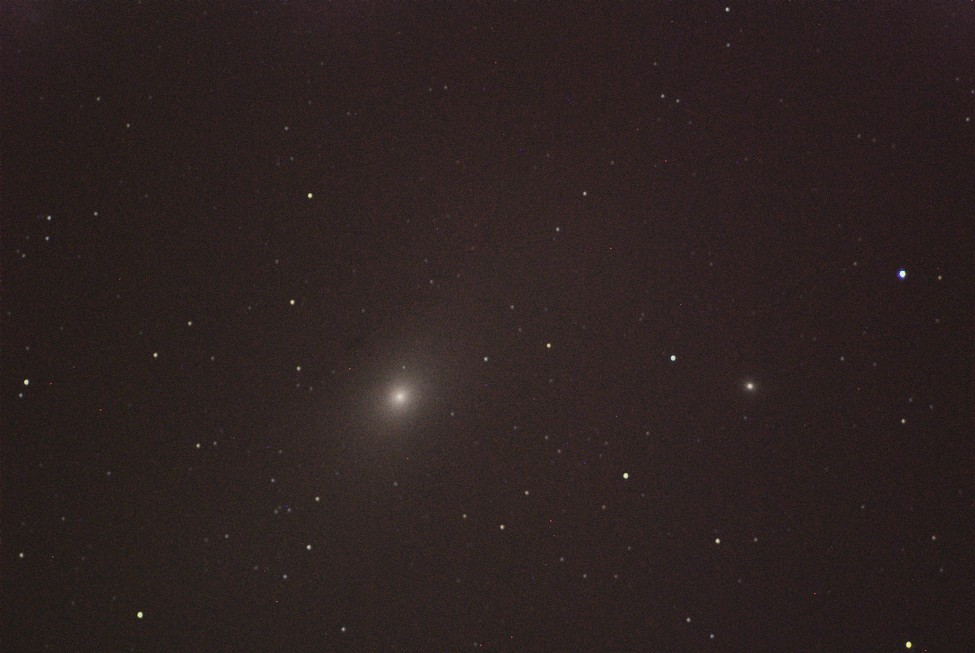In summary, the evening went well even though it was quite chilly.  After getting things up and going I started out by snagging a few, less than impressive, shots of Venus.  Then as it darkened up a bit I set my eyes on getting some better shots of what I could easily find around the night sky.  Especially for my first photography session ever using a DSLR.  I started with M57 the Ring Nebula which was a great site when it popped into view after a simple 4 second exposure!  Once I'd finished here I thought I'd try for something a little more elusive but still a sure thing if I could star hop to it.  Sure enough I was able to get M31 the Andromeda Galaxy as well as one of its companion galaxies (M32) in the frame.  I managed to capture several really good shots with most of them (before processing in some cases) showing the dust lines of the galaxy's spiral arms.  I did not expect to see this detail.  After these I headed for one last, easy to snag, object, M13 the Hercules Globular Cluster which my mount failed to properly find earlier in the night.  Once I star hopped to it I was able to snag some really great shots of it before calling it a night and comping back here to process and post the results.  Hopefully you guys can enjoy the images as much as I enjoyed taking them!

## June 5th 2012 Venus Transit

Rounding off a three-fer in Astronomical events of late is the June 5th 2012 Venus transit across the face of the Sun.

Included here is an approximation of what you should see viewing the sun from Lawrence, KS at approximately 7PM local time and an accompanying video of the transit progress from 5PM local time through sunset.

Transit Image: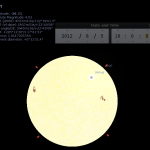While this event may not seem as spectacular as the previous two eclipses (one solar on May 20th and one lunar on June 4th) this event is actually more significant because it is the very last time Venus will cross the face of the sun in our lifetime (unless we really have some astonishing medical accomplishments in the next 30-50 or so years)! The next transit will happen in the year 2117!

I'm also including a site with various viewing information here: http://www.transitofvenus.org

Again, please be safe when viewing this event. You should take the same precautions as you would for a solar eclipse or any other solar viewing event. The site above has a good section on eye safety so please view it in leiu of me regurgitating it here.

Happy viewing and be safe!

## Orbital Period Calculations

*This post re-published & paraphrased from cache – September 30th 2009*

Since this is something that I recently had to do for a game that I’m a developer on, I thought it would be prudent to put together a write-up on the process of determining orbital period of a body around a central mass; specifically sidereal period.  Overall, this is a fairly simple and straight forward problem, but, even so, it is certainly one that every Physics and Astronomy major and enthusiast should be familiar with.

Now, it should be noted that if an object of significant mass is orbiting a central mass, it is probably a good idea to include both masses in the calculation since it could change the result somewhat significantly.  In order to perform this calculation we need to know the following:

•, the gravitational constant
•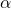, semi-major axis (orbital radius or distance from object 1’s center to object 2’s center)
•, the mass of the central object
•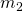, the mass of the orbiting object

So, with this information we can derive a formula from Kepler’s Third Law of motion; the square of the orbital period of a planet is directly proportional to the cube of the semi-major axis of it’s orbit.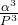and Newton’s Law of Universal Gravitation; attractions are inversely proportional to the squares of the distances between massive bodies.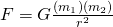Now, the formulas need to be combined to achieve the following relationship: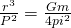The resultant formula can now be manipulated into solving for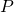or the period of the orbit as follows:Important Note: Since we are using the total mass of the combined bodies we do not multiply the masses for this equation, if calculating the period for 2 large bodies the masses should be added together.

Now that we have our formula, it’s time to work through our information.  The central star is a Type A IV star at ~10,000K with a mass of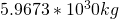.  The planet has a mass of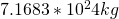and an orbital radius of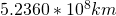.  Next we take this information, along with the Gravitational Constant (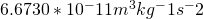and work through our formula:Now combine values and convert the orbital radius into meters so our units match: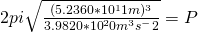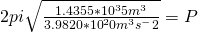Now solve the rest: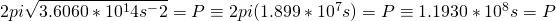Great! Now we have our answer in seconds which means we can convert this number into years to obtain the yearly orbital comparison (the typical way to express orbital periods when it comes to astronomy).  To do this we take our value, divide by 60 for the 60 seconds in a minute, by 60 again for minutes in an hour, by 24 for the hours in a day and then 365 for the days in a year.

[/math]1.1930*10^8s/60s/60m/24h/365d = 3.7830 years[/math]

And there you have it, our answer for the orbital period of the planet is 3.7830 times longer than that of Earths, or 3.7830 years.

In working through this I think there are a few common problems that can happen, so if you attempt this calculation and do not arrive at an answer you would expect, make sure to check the following things:

• Make sure orbital period gets converted into meters at some point.  The Gravitational Constant is calculated in meters which means your orbital radius value should be as well.
• Make sure to add the masses of the bodies.  This is important to note because other similar formulas (such as in calculating weight on another planet) masses are multiplied.  This calculation uses total combined mass.
• Check your units.  If your calculations are complete but you did not net up with seconds for a unit your calculations could be flawed.

In closing, please let me know if you find any flaws with this write-up.  I have put it together carefully, however, I am not immune to mistakes and have corrected several so far.

## How to Calculate Weight on Another Planet

*This post re-published from cache – August 28th 2009*

In working on answering a question today, I was rather perturbed by the fact that I was not able to find a definitive answer to a question that I felt should be well defined.  That question was: If I weigh 100lbs on Earth, how much would I weigh on Saturn?

Simple question right?  Not by what I found online.  There are a number of different sites that can be used to get a quick ( and possibly incorrect) answer.  I found the sites that I visited in search of an answer to be at the least, inaccurate and at the most, dead wrong.  The answers ranged from around 55lbs to about 115lbs.  On top of this, the method for calculating weight on another planet was based on the gravitational acceleration at the surface.  This is something that is defined already depending on the site or information you look at, but again, the values varied significantly.

Here’s an easy way to do it and get a reliable answer:

Find the gravitational acceleration, or more specifically the ratio of acceleration in relation to Earth, where Earth is a value of 1.  Now, find a table online with the planet’s ratio that you want to calculate.  For Saturn I used 1.065 (1.07 significant digits) found here.  Simply cross-multiply to get your answer: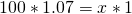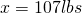Now that we have the easy answer I think it is important to understand that without already having the gravitational ratio this is still calculable by obtaining the gravitational ratio on our own, as shown below.

Before we begin, it should be noted that the radius of a planet type body varies from point-to-point.  This means that your answer will also vary depending on where you measure from.  This could account for some of the discrepancies that I found on other sites.  For example, you will be heavier at either of the poles and lighter at the equator.  This is due to the bulge at the equator caused by rotation.  This increases distance from the center of mass and reduces the gravitational forces on you slightly, causing you to weigh less.  For our purposes, we will use the mean radius vs. a specific measurement.

First, we need my mass in kilograms (kg), rather than weight in pounds (lbs) so we use the formula here: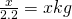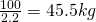Great, now that we have the mass, we can move forward.  We will need additional information at this point.  The formula that will allow us to make further calculations, Newton’s Law of Universal Gravitation.It should be noted that the value forshould be in meters, the values ofandin kilograms and the resultant value of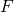will be in Newtons.

This formula will allow us to calculate the force exerted between objects of a given mass, at a given distance from the center of gravity.  In turn, allowing us to calculate the respective weight.  For this formula to work, we will need additional statistics.  Since we are calculating for Saturn specifically, I took the values found from the NASA stats page linked earlier.

•= The Gravitational Constant (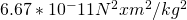)
•= Object 1 Mass, Saturn ()
•= Object 2 Mass, Person (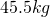)
•= Mean Radius, Saturn (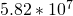)

Now that we have all the variables needed, we can use Newton’s formula to get the result: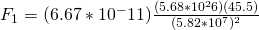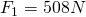Now we have the gravitational force (F) for Saturn.  In order to make a comparison based on weight, we will also need to do the same calculations for Earth.  Since we already have the gravitational constant and our calculated mass, we need to acquire Earth’s mean radius and it’s mass in kilograms.  These can both be found through the site linked earlier.

•= The Gravitational Constant ()
•= Object 1 Mass, Earth (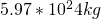)
•= Object 2 Mass, Person ()
•= Mean Radius, Earth (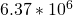)

Once again we can use Newton’s formula to calculate the value of F: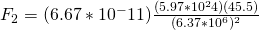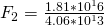Now that we have both values we can compute the ratio: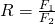where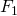is the value of Saturn and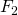is Earth.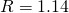Curiously enough, the ratio arrived at in this equation is higher than the 1.065 cited in the NASA document.  I am assuming this is due to differences in the value used for each planet’s radius.  I will see what I can do about further investigating this discrepancy and amend my post if needed.

Now, to finish our process.  We once again cross-multiply using 100lbs to 1 for the value on Earth.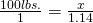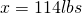In conclusion, the variations in answer can be explained by taking into account the differences in radius values used in the calculations.  Polar radius, Equatorial radius and mean radius will all give values slightly different from one another in a difference of.

With our calculations it can be assumed that we have a possibility for error.  Based on this information and given a discrepancy of a significant 7.4 lbs, this could account for roughly 7-13% variance in answers to this question.  That said, make certain to note which radius measurement is being asked for when calculating these types of problems.  It could mean the difference between a right and wrong answer.